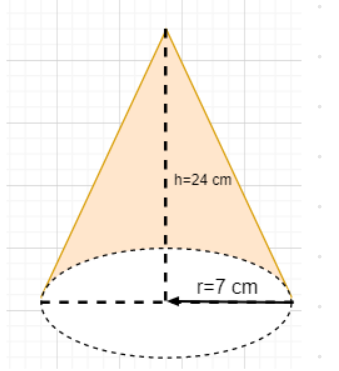Courses
Courses for Kids
Free study material
Free LIVE classes
More# A joker’s cap is in the form of a cone of radius 7 cm and height 24 cm. Find the area of the cardboard required to make the cap.Last updated date: 20th Mar 2023
Total views: 307.5k
Views today: 4.86kVerified
Given, radius of the cone-shaped joker’s cap $r = 7{\text{ cm}}$
Height of the cone-shaped joker’s cap $h = 24{\text{ cm}}$
As we know that the curved surface area of the cone with radius as $r$ and height as $h$ is given by $\pi rl$ where slant height is $l = \sqrt {{r^2} + {h^2}}$
$\Rightarrow$Area of the cardboard required to make the cap$=$Curved surface area of the cap$= \pi rl = \pi r\sqrt {{r^2} + {h^2}} = \dfrac{{22}}{7} \times 7 \times \sqrt {{7^2} + {{24}^2}} = 22 \times 25 = 550{\text{ c}}{{\text{m}}^2}$